# AP Physics C: Electricity and Magnetism Practice Test 8

### Test Information11 questions15 minutes

1.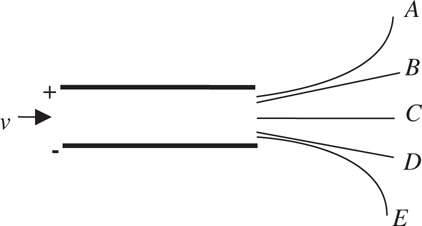An electron moving at constant velocity enters the region between two charged plates, as shown above. Which of the paths above correctly shows the electron’s trajectory after leaving the region between the charged plates?

2. Two isolated particles, A and B, are 4 m apart. Particle A has a net charge of 2Q, and B has a net charge of Q. The ratio of the magnitude of the electric force on A to that on B is

3.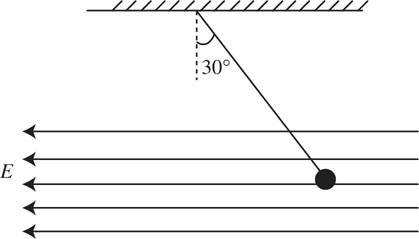A uniform electric field points to the left. A small metal ball charged to −2 mC hangs at a 30° angle from a string of negligible mass, as shown above. The tension in the string is measured to be 0.1 N. What is the magnitude of the electric field? (sin 30° = 0.50; cos 30° = 0.87; tan 30° = 0.58.)

4.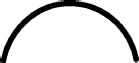A thin semicircular conductor of radius R holds charge +Q. What is the magnitude and direction of the electric field at the center of the circle?

5. Above an infinitely large plane carrying charge density σ, the electric field points up and is equal to σ/2εo. What is the magnitude and direction of the electric field below the plane?

6.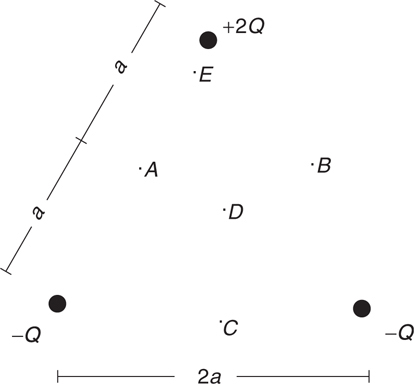Three charges are arranged in an equilateral triangle, as shown above. At which of these points is the electric potential smallest?

7.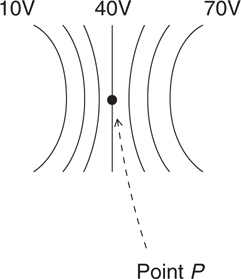The diagram shows a set of equipotential surfaces. At point P, what is the direction of the electric field?

8. A metal sphere carries charge Q; a nonconducting sphere of equal size carries the same charge Q, uniformly distributed throughout the sphere. These spheres are isolated from each other. Consider the electric field at the center of the spheres, within the spheres, and outside the spheres. Which of these electric fields will be the same for both spheres, and which will be different?

9. Under what conditions is the net electric flux through a closed surface proportional to the enclosed charge?

10.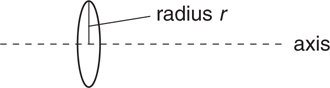A hollow metal ring of radius r carries charge q. Consider an axis straight through the center of the ring. At what point(s) along this axis is/are the electric field equal to zero?

11.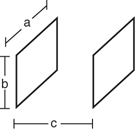A parallel plate capacitor consists of identical rectangular plates of dimensions a × b, separated by a distance c. To cut the capacitance of this capacitor in half, which of these quantities should be doubled?